## 2.6Forces at a surface

The next law to deﬁne is that of conservation of momentum, i.e. Newton’s second law of motion (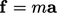) for ﬂuids. It involves forces within the ﬂuid so requires a description of forces at a surface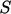bounding a volume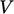.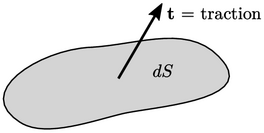The force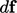is in the direction of the traction vector with magnitude of (traction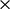surface area) — compare with Eq. (2.3 )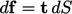(2.16)
The tractions at a surface bounding some volume of ﬂuid (or any continuum, e.g. solid) depend on the orientation of the surface. Consequently, we cannot deﬁne forces within a ﬂuid as traction vectors at points within the ﬂuid.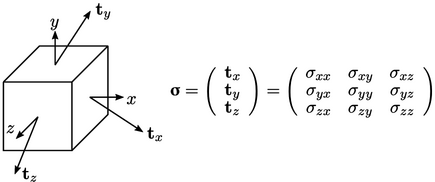Instead, we require 3 traction vectors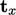,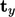and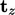, deﬁned in planes perpendicular to one another, i.e.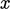,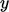and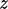. This results in the stress tensor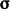with 9 components, consisting of 3 traction vectors, each containing 3 components.

The traction can be calculated at a surface with any orientation by taking the inner product of the unit normal vector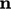and the stress tensorsuch that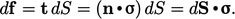(2.17)

### Tensors

We have deﬁned the stress tensor3, an entity with 9 component values, corresponding to our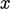,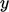and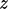axes (or more speciﬁcally base vectors of unit length aligned with our,andaxes).

In fact, the term “tensor” describes any entity with multiple component values corresponding to the dimensions of space — here 3. A tensor has rank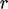, such that the number of component values for 3D space =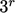.

In this book we use the term “tensor” to mean “tensor of rank 2” unless otherwise noted. A vector is a tensor of rank 1 and a scalar is rank 0.

The inner product of a vector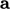and tensor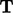produces a vector whose 3 components are evaluated as follows: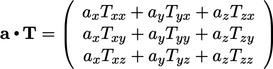(2.18)
This inner product tensor is commutative only if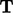is symmetric since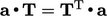— see Eq. (2.24 ) for the transpose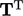.
3More precisely the “Cauchy stress tensor”, introduced by Augustin-Louis Cauchy in De la pression ou tension dans un corps solide, 1827.

Notes on CFD: General Principles - 2.6 Forces at a surface